# 2.3 Properties of the real numbers  (Page 2/2)

 Page 2 / 2

$\begin{array}{cc}\left(9y\right)4=9\left(y4\right)& \text{Both}\text{\hspace{0.17em}}\text{represent}\text{\hspace{0.17em}}\text{the}\text{\hspace{0.17em}}\text{same}\text{\hspace{0.17em}}\text{product}\text{.}\end{array}$

## Practice set b

Fill in the $\left(\begin{array}{cc}& \end{array}\right)$ to make each statement true. Use the associative properties.

$\left(9+2\right)+5=9+\left(\begin{array}{cc}& \end{array}\right)$

$2+5$

$x+\left(5+y\right)=\left(\begin{array}{cc}& \end{array}\right)+y$

$x+5$

$\left(11a\right)6=11\left(\begin{array}{cc}& \end{array}\right)$

$a\cdot 6$

$\left[\left(7m-2\right)\left(m+3\right)\right]\left(m+4\right)=\left(7m-2\right)\left[\left(\begin{array}{cc}& \end{array}\right)\left(\begin{array}{cc}& \end{array}\right)\right]$

$\left(m+3\right)\left(m+4\right)$

## Sample set c

Simplify (rearrange into a simpler form): $5x6b8ac4$ .

According to the commutative property of multiplication, we can make a series of consecutive switches and get all the numbers together and all the letters together.

$\begin{array}{ll}5\cdot 6\cdot 8\cdot 4\cdot x\cdot b\cdot a\cdot c\hfill & \hfill \\ 960xbac\hfill & \text{Multiply}\text{\hspace{0.17em}}\text{the}\text{\hspace{0.17em}}\text{numbers}\text{.}\hfill \\ 960abcx\hfill & \text{By}\text{\hspace{0.17em}}\text{convention,}\text{\hspace{0.17em}}\text{we}\text{\hspace{0.17em}}\text{will,}\text{\hspace{0.17em}}\text{when}\text{\hspace{0.17em}}\text{possible,}\text{\hspace{0.17em}}\text{write}\text{\hspace{0.17em}}\text{all}\text{\hspace{0.17em}}\text{letters}\text{\hspace{0.17em}}\text{in}\text{\hspace{0.17em}}\text{alphabetical}\text{\hspace{0.17em}}\text{order}\text{.}\hfill \end{array}$

## Practice set c

Simplify each of the following quantities.

$3a7y9d$

$189ady$

$6b8acz4\cdot 5$

$960abcz$

$4p6qr3\left(a+b\right)$

$72pqr\left(a+b\right)$

## The distributive properties

When we were first introduced to multiplication we saw that it was developed as a description for repeated addition.

$4+4+4=3\cdot 4$

Notice that there are three 4’s, that is, 4 appears 3 times . Hence, 3 times 4.
We know that algebra is generalized arithmetic. We can now make an important generalization.

When a number $a$ is added repeatedly $n$ times, we have
$\underset{a\text{\hspace{0.17em}}\text{appears}\text{\hspace{0.17em}}n\text{\hspace{0.17em}}\text{times}}{\underbrace{a+a+a+\cdots +a}}$
Then, using multiplication as a description for repeated addition, we can replace
$\begin{array}{ccc}\underset{n\text{\hspace{0.17em}}\text{times}}{\underbrace{a+a+a+\cdots +a}}& \text{with}& na\end{array}$

For example:

$x+x+x+x$ can be written as $4x$ since $x$ is repeatedly added 4 times.

$x+x+x+x=4x$

$r+r$ can be written as $2r$ since $r$ is repeatedly added 2 times.

$r+r=2r$

The distributive property involves both multiplication and addition. Let’s rewrite $4\left(a+b\right).$ We proceed by reading $4\left(a+b\right)$ as a multiplication: 4 times the quantity $\left(a+b\right)$ . This directs us to write

$\begin{array}{lll}4\left(a+b\right)\hfill & =\hfill & \left(a+b\right)+\left(a+b\right)+\left(a+b\right)+\left(a+b\right)\hfill \\ \hfill & =\hfill & a+b+a+b+a+b+a+b\hfill \end{array}$

Now we use the commutative property of addition to collect all the $a\text{'}s$ together and all the $b\text{'}s$ together.

$\begin{array}{lll}4\left(a+b\right)\hfill & =\hfill & \underset{4a\text{'}s}{\underbrace{a+a+a+a}}+\underset{4b\text{'}s}{\underbrace{b+b+b+b}}\hfill \end{array}$

Now, using multiplication as a description for repeated addition, we have

$\begin{array}{lll}4\left(a+b\right)\hfill & =\hfill & 4a+4b\hfill \end{array}$

We have distributed the 4 over the sum to both $a$ and $b$ .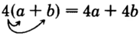## The distributive property

$\begin{array}{cc}a\left(b+c\right)=a\cdot b+a\cdot c& \left(b+c\right)\end{array}a=a\cdot b+a\cdot c$

The distributive property is useful when we cannot or do not wish to perform operations inside parentheses.

## Sample set d

Use the distributive property to rewrite each of the following quantities.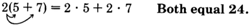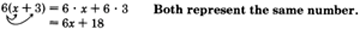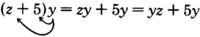## Practice set d

What property of real numbers justifies
$a\left(b+c\right)=\left(b+c\right)a?$

the commutative property of multiplication

Use the distributive property to rewrite each of the following quantities.

$3\left(2+1\right)$

$6+3$

$\left(x+6\right)7$

$7x+42$

$4\left(a+y\right)$

$4a+4y$

$\left(9+2\right)a$

$9a+2a$

$a\left(x+5\right)$

$ax+5a$

$1\left(x+y\right)$

$x+y$

## The identity properties

The number 0 is called the additive identity since when it is added to any real number, it preserves the identity of that number. Zero is the only additive identity.
For example, $6+0=6$ .

## Multiplicative identity

The number 1 is called the multiplicative identity since when it multiplies any real number, it preserves the identity of that number. One is the only multiplicative identity.
For example $6\cdot 1=6$ .

We summarize the identity properties as follows.

$\begin{array}{cc}\begin{array}{l}\text{ADDITIVE}\text{\hspace{0.17em}}\text{IDENTITY}\\ \text{\hspace{0.17em}}\text{\hspace{0.17em}}\text{\hspace{0.17em}}\text{\hspace{0.17em}}\text{\hspace{0.17em}}\text{\hspace{0.17em}}\text{\hspace{0.17em}}\text{\hspace{0.17em}}\text{\hspace{0.17em}}\text{PROPERTY}\end{array}& \begin{array}{l}\text{MULTIPLICATIVE}\text{\hspace{0.17em}}\text{IDENTITY}\\ \text{​}\text{​}\text{​}\text{​}\text{​}\text{\hspace{0.17em}}\text{\hspace{0.17em}}\text{\hspace{0.17em}}\text{\hspace{0.17em}}\text{\hspace{0.17em}}\text{\hspace{0.17em}}\text{\hspace{0.17em}}\text{\hspace{0.17em}}\text{\hspace{0.17em}}\text{\hspace{0.17em}}\text{\hspace{0.17em}}\text{\hspace{0.17em}}\text{\hspace{0.17em}}\text{\hspace{0.17em}}\text{\hspace{0.17em}}\text{\hspace{0.17em}}\text{\hspace{0.17em}}\text{\hspace{0.17em}}\text{\hspace{0.17em}}\text{PROPERTY}\end{array}\\ \text{If}\text{\hspace{0.17em}}a\text{\hspace{0.17em}}\text{is}\text{\hspace{0.17em}}\text{a}\text{\hspace{0.17em}}\text{real}\text{\hspace{0.17em}}\text{number,\hspace{0.17em}then}& \text{If}\text{\hspace{0.17em}}a\text{\hspace{0.17em}}\text{is}\text{\hspace{0.17em}}\text{a}\text{\hspace{0.17em}}\text{real}\text{\hspace{0.17em}}\text{number,}\text{\hspace{0.17em}}\text{then}\\ a+0=a\text{\hspace{0.17em}}\text{\hspace{0.17em}}\text{\hspace{0.17em}}\text{\hspace{0.17em}}\text{\hspace{0.17em}}\text{\hspace{0.17em}}\text{\hspace{0.17em}}\text{\hspace{0.17em}}\text{\hspace{0.17em}}\text{and}\text{\hspace{0.17em}}\text{\hspace{0.17em}}\text{\hspace{0.17em}}\text{\hspace{0.17em}}\text{\hspace{0.17em}}\text{\hspace{0.17em}}\text{\hspace{0.17em}}\text{\hspace{0.17em}}0+a=a& a\cdot 1=a\text{\hspace{0.17em}}\text{\hspace{0.17em}}\text{\hspace{0.17em}}\text{\hspace{0.17em}}\text{\hspace{0.17em}}\text{\hspace{0.17em}}\text{\hspace{0.17em}}\text{\hspace{0.17em}}\text{and}\text{\hspace{0.17em}}\text{\hspace{0.17em}}\text{\hspace{0.17em}}\text{\hspace{0.17em}}\text{\hspace{0.17em}}\text{\hspace{0.17em}}\text{\hspace{0.17em}}\text{\hspace{0.17em}}1\cdot a=a\end{array}$

## The inverse properties

When two numbers are added together and the result is the additive identity, 0, the numbers are called additive inverses of each other. For example, when 3 is added to $-3$ the result is 0, that is, $3+\left(-3\right)=0$ . The numbers 3 and $-3$ are additive inverses of each other.

## Multiplicative inverses

When two numbers are multiplied together and the result is the multiplicative identity, 1, the numbers are called multiplicative inverses of each other. For example, when 6 and $\frac{1}{6}$ are multiplied together, the result is 1, that is, $6\cdot \frac{1}{6}=1$ . The numbers 6 and $\frac{1}{6}$ are multiplicative inverses of each other.

We summarize the inverse properties as follows.

## The inverse properties

1. If $a$ is any real number, then there is a unique real number $-a$ , such that
$\begin{array}{ccc}a+\left(-a\right)=0& \text{and}& -a+a=0\end{array}$
The numbers $a$ and $-a$ are called additive inverses of each other.
2. If $a$ is any nonzero real number, then there is a unique real number $\frac{1}{a}$ such that
$\begin{array}{ccc}a\cdot \frac{1}{a}=1& \text{and}& \frac{1}{a}\end{array}\cdot a=1$
The numbers $a$ and $\frac{1}{a}$ are called multiplicative inverses of each other.

## Expanding quantities

When we perform operations such as $6\left(a+3\right)=6a+18$ , we say we are expanding the quantity $6\left(a+3\right)$ .

## Exercises

Use the commutative property of addition and multiplication to write expressions for an equal number for the following problems. You need not perform any calculations.

$x+3$

$3+x$

$5+y$

$10x$

$10x$

$18z$

$r6$

$6r$

$ax$

$xc$

$cx$

$7\left(2+b\right)$

$6\left(s+1\right)$

$\left(s+1\right)6$

$\left(8+a\right)\left(x+6\right)$

$\left(x+16\right)\left(a+7\right)$

$\left(a+7\right)\left(x+16\right)$

$\left(x+y\right)\left(x-y\right)$

$0.06m$

$m\left(0.06\right)$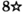$5\left(6h+1\right)$

$\left(6h+1\right)5$

$m\left(a+2b\right)$

$k\left(10a-b\right)$

$\left(10a-b\right)k$

$\left(21c\right)\left(0.008\right)$

$\left(-16\right)\left(4\right)$

$\left(4\right)\left(-16\right)$

$\left(5\right)\left(b-6\right)$

$\square \text{\hspace{0.17em}}\cdot ○$

$○\text{\hspace{0.17em}}\cdot \text{\hspace{0.17em}}\square$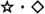Simplify using the commutative property of multiplication for the following problems. You need not use the distributive property.

$9x2y$

$18xy$

$5a6b$

$2a3b4c$

$24abc$

$5x10y5z$

$1u3r2z5m1n$

$30mnruz$

$6d4e1f2\left(g+2h\right)$

$\left(\frac{1}{2}\right)d\left(\frac{1}{4}\right)e\left(\frac{1}{2}\right)a$

$\frac{1}{16}ade$

$3\left(a+6\right)2\left(a-9\right)6b$

$1\left(x+2y\right)\left(6+z\right)9\left(3x+5y\right)$

$9\left(x+2y\right)\left(6+z\right)\left(3x+5y\right)$

For the following problems, use the distributive property to expand the quantities.

$2\left(y+9\right)$

$b\left(r+5\right)$

$br+5b$

$m\left(u+a\right)$

$k\left(j+1\right)$

$jk+k$

$x\left(2y+5\right)$

$z\left(x+9w\right)$

$xz+9wz$

$\left(1+\text{\hspace{0.17em}}d\right)e$

$\left(8+\text{\hspace{0.17em}}2f\right)g$

$8g+2fg$

$c\left(2a+\text{\hspace{0.17em}}10b\right)$

$15x\left(2y+\text{\hspace{0.17em}}3z\right)$

$30xy+45xz$

$8y\left(12a+b\right)$

$z\left(x+y+m\right)$

$xz+yz+mz$

$\left(a+6\right)\left(x+y\right)$

$\left(x+10\right)\left(a+b+c\right)$

$ax+bx+cx+10a+10b+10c$

$1\left(x+y\right)$

$1\left(a+16\right)$

$a+16$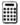$0.48\left(0.34a+0.61\right)$$21.5\left(16.2a+3.8b+0.7c\right)$

$348.3a+81.7b+15.05c$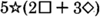$2{z}_{t}\left({L}_{m}+8k\right)$

$2{L}_{m}{z}_{t}+16k{z}_{t}$

## Exercises for review

( [link] ) Find the value of $4\cdot 2+5\left(2\cdot 4-6÷3\right)-2\cdot 5$ .

( [link] ) Is the statement $3\left(5\cdot 3-3\cdot 5\right)+6\cdot 2-3\cdot 4<0$ true or false?

false

( [link] ) Draw a number line that extends from $-2$ to 2 and place points at all integers between and including $-2$ and 3.

( [link] ) Replace the $\ast$ with the appropriate relation symbol $\left(<,>\right).-7\ast -3$ .

$<$

( [link] ) What whole numbers can replace $x$ so that the statement $-2\le x<2$ is true?

a diagram of an adult mosquito
what are white blood cells
white blood cell is part of the immune system. that help fight the infection.
MG
Mlungisi
Cells with a similar function, form a tissue. For example the nervous tissue is composed by cells:neurons and glia cells. Muscle tissue, is composed by different cells.
Anastasiya
I need further explanation coz celewi anything guys,,,
hey guys
Isala
on what?
Anastasiya
is air homogenous or hetrogenous
homogenous
Kevin
why saying homogenous?
Isala
explain if oxygen is necessary for photosynthesis
explain if oxygen is necessary for photosynthesis
Yes, the plant does need oxygen. The plant uses oxygen, water, light, and produced food. The plant use process called photosynthesis.
MG
By using the energy of sunlight, plants convert carbon dioxide and water into carbohydrates and oxygen by photosynthesis. This happens during the day and sunlight is needed.
NOBLE
no. it s a product of the process
Anastasiya
yet still is it needed?
NOBLE
no. The reaction is: 6CO2+6H20+ solar energy =C6H12O6(glucose)+602. The plant requires Carbon dioxyde, light, and water Only, and produces glucose and oxygen( which is a waste).
Anastasiya
what was the question
joining
Godfrey
the specific one
NOBLE
the study of non and living organism is called.
Godfrey
Is call biology
Alohan
yeah
NOBLE
yes
Usher
what Is ecology
what is a cell
A cell is a basic structure and functional unit of life
Ndongya
what is biolgy
is the study of living and non living organisms
Ahmed
may u draw the female organ
i dont understand
Asal
:/
Asal
me too
DAVID
anabolism and catabolism
Anabolism refers to the process in methabolism in which complex molecules are formed "built" and requires energy to happen. Catabolism is the opposite process: complex molecules are deconstructed releasing energy, such as during glicolysis.
Anastasiya
Explain briefly independent assortment gene .
hi
Amargo
hi I'm Anatalia
Joy
what do you mean by pituitary gland
Digambar
draw and lable the cell
why homones are destroyed soon after completing their role
Got questions? Join the online conversation and get instant answers!ByByBy Yasser IbrahimBy Sam LuongBy OpenStaxBy RhodesBy OpenStaxBy Jonathan LongBy JavaChamp TeamBy Madison ChristianBy OpenStaxBy OpenStax